# High School Chemistry : Using Gay-Lussac's Law

## Example Questions

### Example Question #1 : Using Gay Lussac's Law

What happens to the pressure of a closed gaseous system when the temperature increases two-fold?

The pressure is halved

The pressure stays the name

The pressure is quartered

The pressure is doubled

The pressure is doubled

Explanation:

To solve this question, we will need to use Gay-Lussac's Law: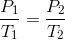This law shows a direct relationship between temperature and pressure: as temperature increases, pressure will increase proportionally. Mathematically, we can solve for the change in pressure by inputting the change in temperature. The second temperature is equal to twice the first temperature. For the fractions to remain equal, the second pressure must also be twice the first pressure.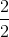### Example Question #1 : Using Gay Lussac's Law

An ideal gas is contained in a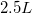container at a pressure of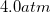. The container is at a temperature of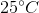.

What will be the final pressure if the temperature is increased to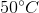?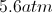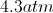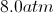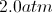Explanation:

Since the only variable that has changed is temperature, we can use Gay-Lussac's law in order to compare pressure to temperature. Because temperature and pressure are on opposite sides of the ideal gas law, they are directly proportional to one another. In other words, as one increases, the other increases as well.

Gay-Lussac's law is written as follows:To use this equation, we must convert the temperature to Kelvin.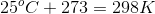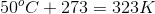Now, use these temperatures and the initial pressure to solve for the final pressure.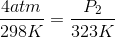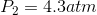### Example Question #21 : Gases And Gas Laws

What law is represented by the following formula?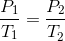Boyle's law

Combined gas law

Charles's law

Gay-Lussac's law

Ideal gas law

Gay-Lussac's law

Explanation:

Gay-Lussac's law defines the direct relationship between temperature and pressure. When the parameters of a system change, Gay-Lussac's law helps us anticipate the effect the changes have on pressure and temperature.Boyle's law relates pressure and volume: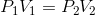Charles's law relates temperature and volume: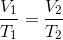The combined gas law takes Boyle's, Charles's, and Gay-Lussac's law and combines it into one law: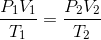The ideal gas law relates temperature, pressure, volume, and moles in coordination with the ideal gas constant: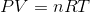### Example Question #2 : Using Gay Lussac's Law

The graph depicted here represents which of the gas laws?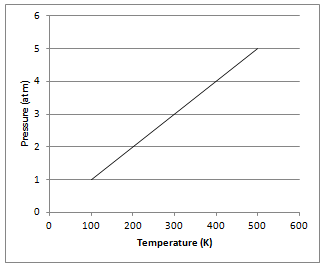Newton's second law

Boyle's law

Charles's law

Gay-Lussac's law

Gay-Lussac's law

Explanation:

The graph shows that gas pressure varies directly with Kelvin temperature at a constant volume, as determined by Gay-Lussac. Gay-Lussac's law can be represented mathematically for a given mass of gas at a constant volume as follows:Boyle's law shows the relationship between pressure and volume. Charles's law shows the relationship between volume and temperature. Newton's second law is not related to gases and shows the relationship between force, mass, and acceleration.

### Example Question #21 : Gases And Gas Laws

A family leaves for summer vacation by driving on the highway. The car’s tires start the trip with a pressure of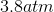at a temperature of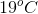and a volume of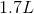. What is the pressure of the tires after driving, when the temperature within the tire increases to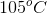?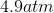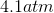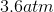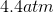Explanation:

We can assume that the volume of the tires remains constant. This allows us to apply Gay-Lussac's law, which related pressure and temperature:We know both the initial and final temperatures, but we must convert to Kelvin in order to use SI units.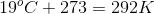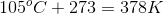Using these temperatures and the initial pressure, we can solve for the final pressure.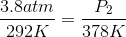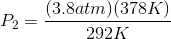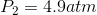### Example Question #1 : Using Gay Lussac's Law

If the temperature of a gas increases, what happens to the pressure?

We must know the molecular formula of the gas to determine the relationship

Pressure will decrease

Pressure will increase

We must know the volume of the gas to determine the relationship

Pressure will remain unchanged

Pressure will increase

Explanation:

According to Gay-Lussac's law, the attributes of pressure and temperature vary by direct proportionality. As temperature increases, pressure increases as well.### Example Question #16 : Gases

If the pressure of a sample of one mole of an ideal gas is increased from 2atm to 3atm at a constant volume, and the initial temperature was 20˚C, what is the final temperature of the sample?

195.3K

303K

439.5K

30K

439.5K

Explanation:

Because the mass and volume of the sample of the ideal gas are kept constant, a change in pressure causes only a direct change in the temperature. This can be derived from the following ideal gas equation:.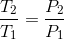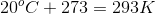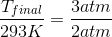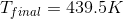### Example Question #1 : Using Gay Lussac's Law

A sample of chlorine gas fills a vessel at a temperature of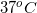. The vessel has a volume of 3L and experiences a pressure of 3atm. What is the mass of the chlorine gas in the vessel?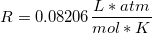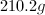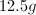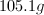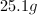Explanation:

This problem requires us to substitute the moles of gas in the ideal gas law to mass over molar mass.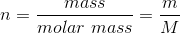We can then isolate for the mass of chlorine gas in the vessel.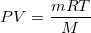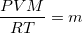Chlorine is diatomic, so its molecular weight will be twice the atomic mass.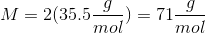The temperature must be expressed in Kelvin.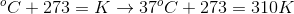Using these values, we can solve for the mass of chlorine gas in the vessel.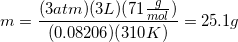### Example Question #7 : Using Gay Lussac's Law

A closed flask contains gas at STP. If the temperature is raised to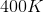what will the pressure be in mmHg?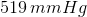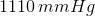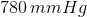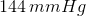Explanation:

Since we have information about temperature and we are interested in changes in pessure, Gay-Lussac's Law will be used. This says thatThe initial temperature and pressure are given (STP) meaning a temperature of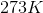and a pressure of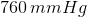. There are other values for standard pressure but they have different units and since the problems requests an answer in units of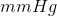we must use the value of standard pressure with those units. Solving for the final pressure gives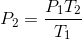Plugging in our variables gives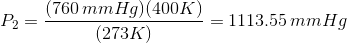We want 3 significant figures since the temperature given has 3, so the final answer is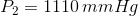### All High School Chemistry Resources